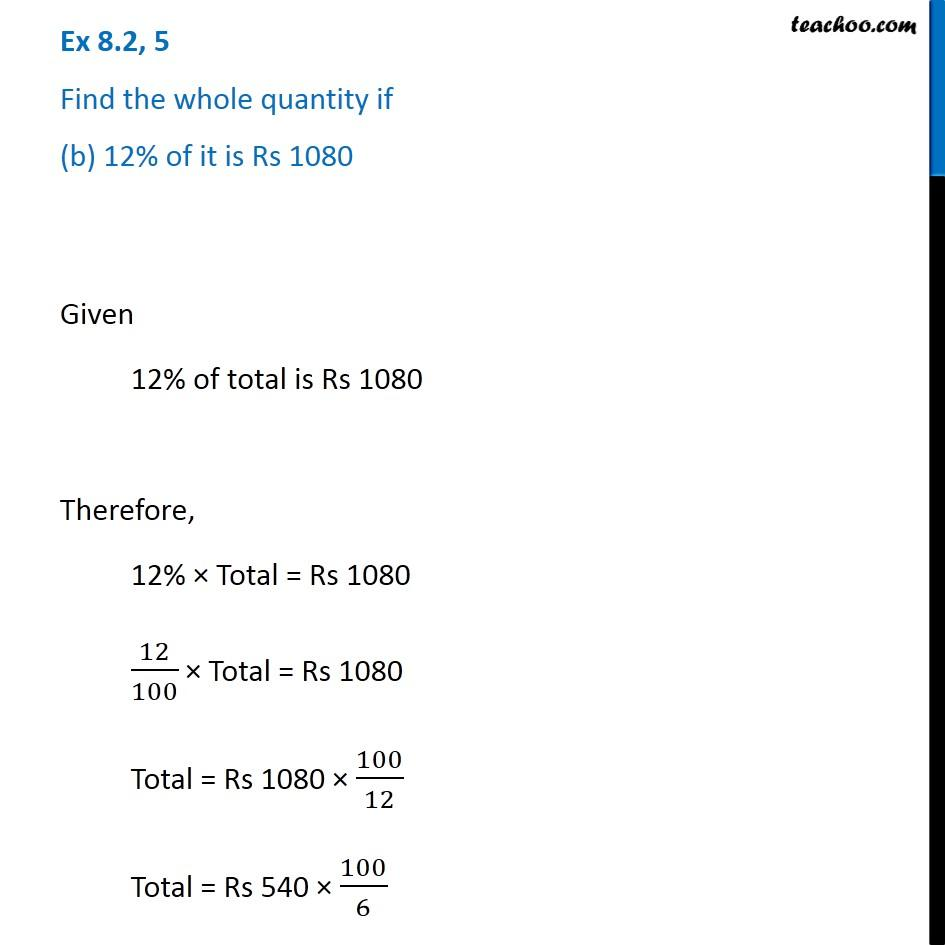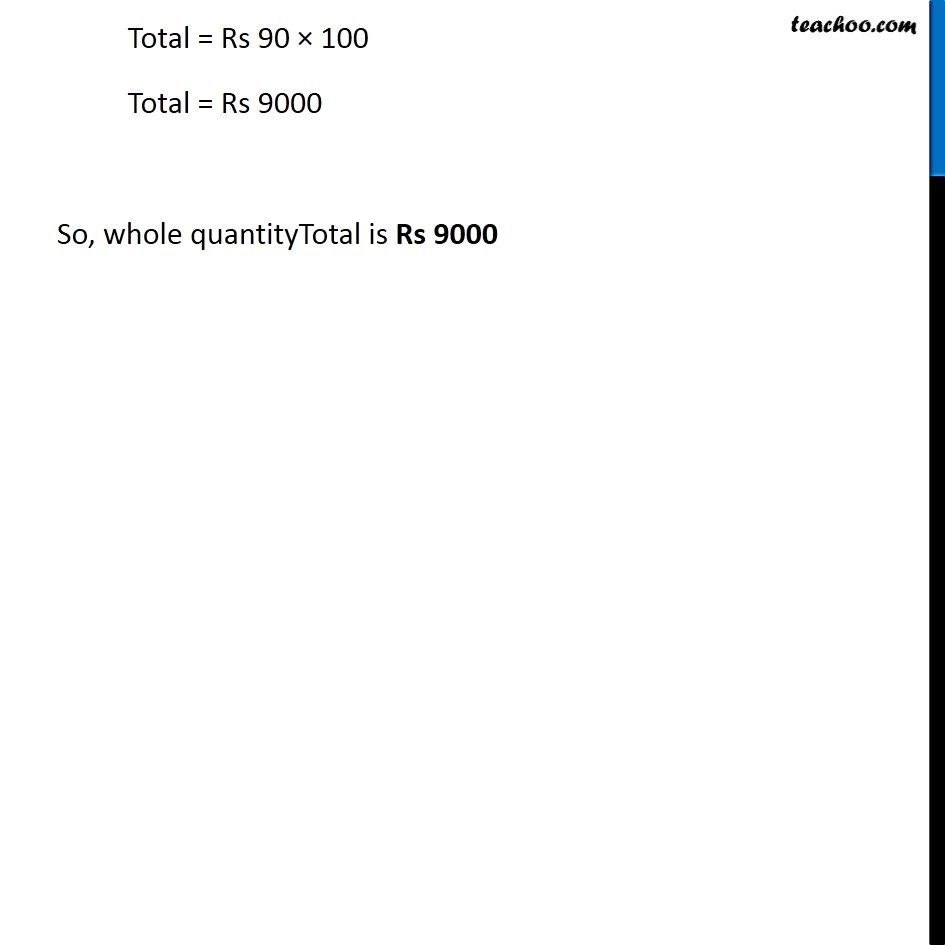Ex 8.2

Chapter 8 Class 7 Comparing Quantities
Serial order wiseGet live Maths 1-on-1 Classs - Class 6 to 12

### Transcript

Ex 8.2, 5 Find the whole quantity if (b) 12% of it is Rs 1080 Given 12% of total is Rs 1080 Therefore, 12% × Total = Rs 1080 12/100 × Total = Rs 1080 Total = Rs 1080 × 100/12 Total = Rs 540 × 100/6 Total = Rs 90 × 100 Total = Rs 9000 So, whole quantityTotal is Rs 9000